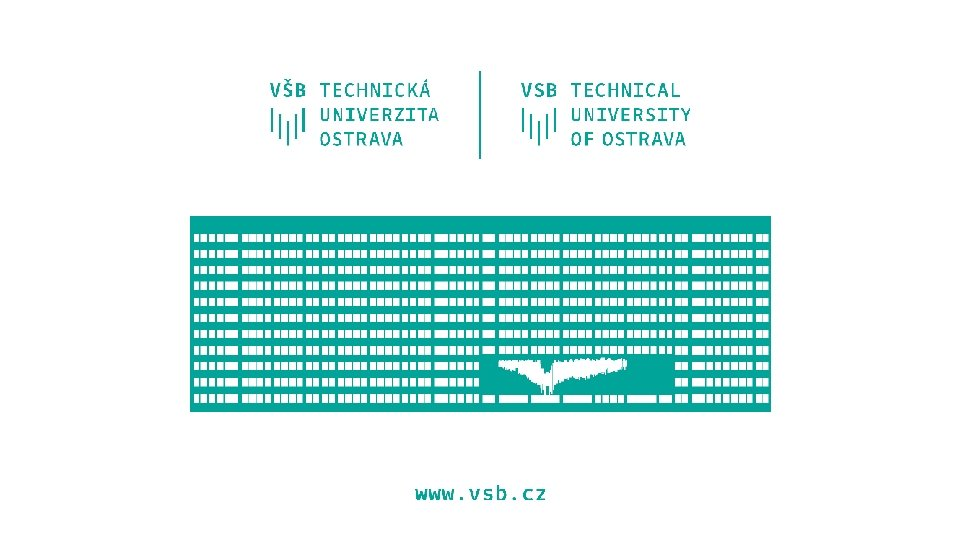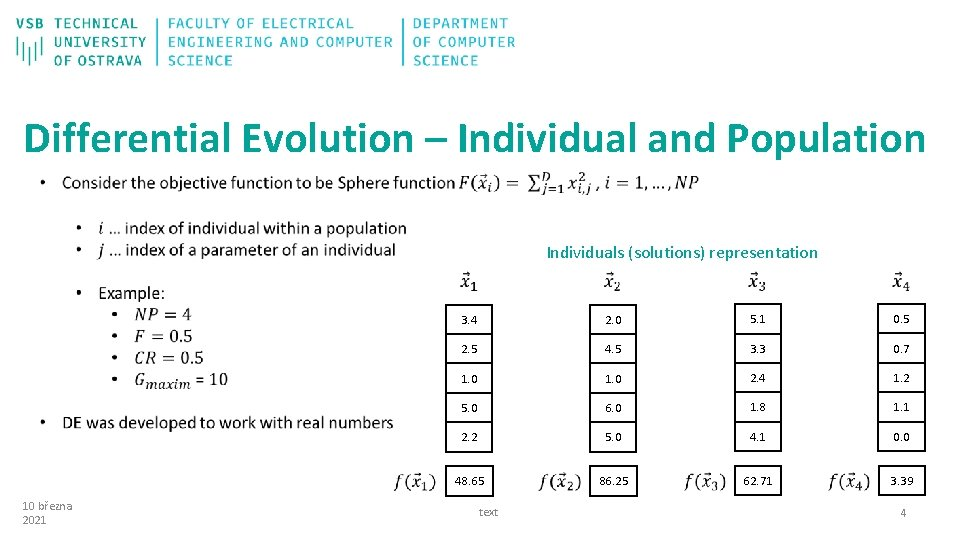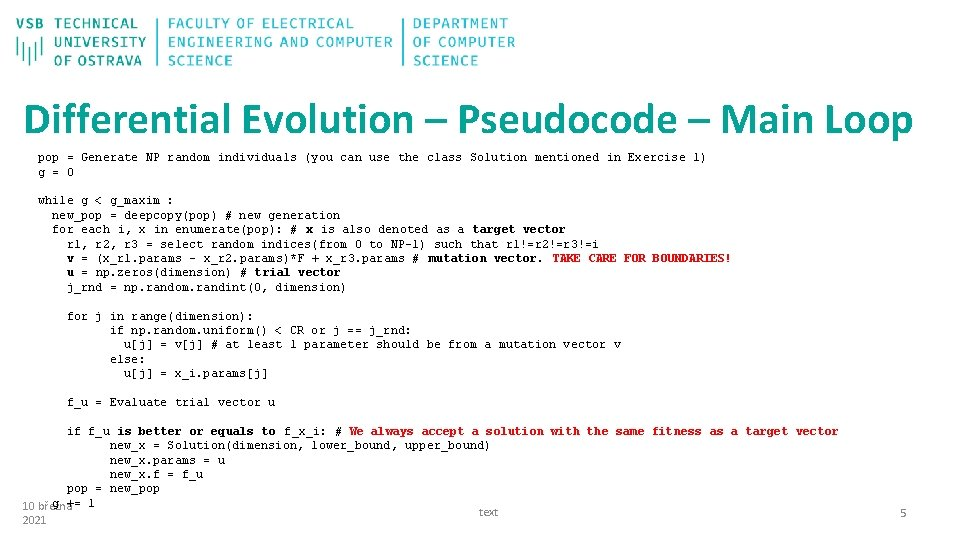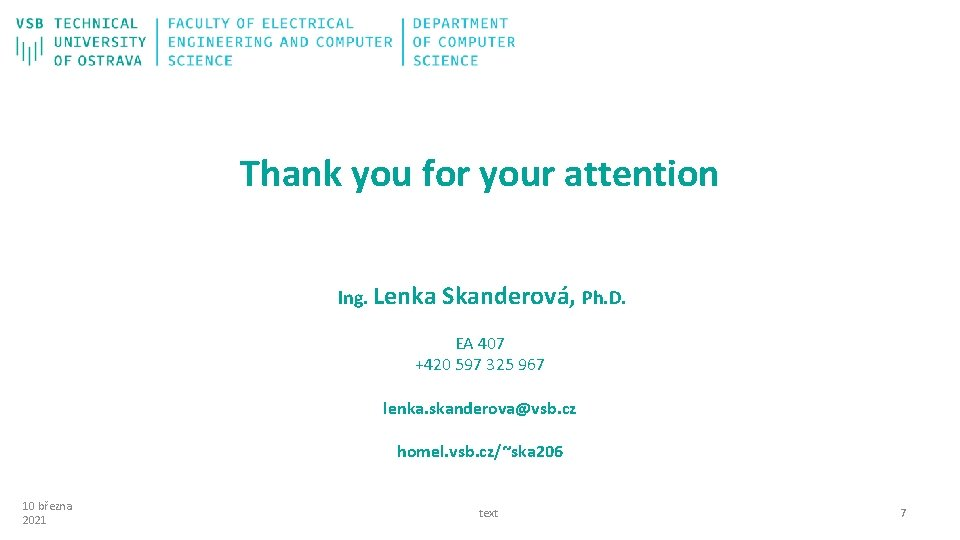# Biologically inspired algorithms Exercise 5 Ing Lenka Skanderov

• Slides: 8
Download presentationBiologically inspired algorithms Exercise 5 Ing. Lenka Skanderová, Ph. D. 10 března 2021 text 1Content • Differential Evolution 10 března 2021 text 2Differential Evolution – Control Parameters 10 března 2021 text 3Differential Evolution – Individual and Population Individuals (solutions) representation 10 března 2021 3. 4 2. 0 5. 1 0. 5 2. 5 4. 5 3. 3 0. 7 1. 0 2. 4 1. 2 5. 0 6. 0 1. 8 1. 1 2. 2 5. 0 4. 1 0. 0 48. 65 text 86. 25 62. 71 3. 39 4Differential Evolution – Pseudocode – Main Loop pop = Generate NP random individuals (you can use the class Solution mentioned in Exercise 1) g = 0 while g < g_maxim : new_pop = deepcopy(pop) # new generation for each i, x in enumerate(pop): # x is also denoted as a target vector r 1, r 2, r 3 = select random indices(from 0 to NP-1) such that r 1!=r 2!=r 3!=i v = (x_r 1. params – x_r 2. params)*F + x_r 3. params # mutation vector. TAKE CARE FOR BOUNDARIES! u = np. zeros(dimension) # trial vector j_rnd = np. random. randint(0, dimension) for j in range(dimension): if np. random. uniform() < CR or j == j_rnd: u[j] = v[j] # at least 1 parameter should be from a mutation vector v else: u[j] = x_i. params[j] f_u = Evaluate trial vector u if f_u is better or equals to f_x_i: # We always accept a solution with the same fitness as a target vector new_x = Solution(dimension, lower_bound, upper_bound) new_x. params = u new_x. f = f_u pop = new_pop g += 10 března 1 text 2021 5Task Figure 2 Figure 1 10 března 2021 Figure 3 text 6Thank you for your attention Ing. Lenka Skanderová, Ph. D. EA 407 +420 597 325 967 lenka. [email protected] cz homel. vsb. cz/~ska 206 10 března 2021 text 7